#Interactive Real Analysis

Next | Previous | Glossary | Map

## 3.3. Subsequences

### Theorem 3.3.4: Bolzano-Weierstrass

Letbe a sequence of real numbers that is bounded. Then there exists a subsequence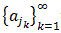that converges.

### Proof:

Since the sequence is bounded, there exists a number M such that | aj | < M for all j. Then:
either [-M, 0] or [0, M] contains infinitely many elements of the sequence
Say that [0, M] does. Choose one of them, and call it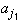either [0, M/2] or [M/2, M] contains infinitely many elements of the (original) sequence.
Say it is [0, M/2]. Choose one of them, and call it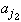either [0, M/4] or [M/4, M/2] contains infinitely many elements of the (original) sequence
This time, say it is [M/4, M/2]. Pick one of them and call it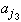Keep on going in this way, halving each interval from the previous step at the next step, and choosing one element from that new interval. Here is what we get:

• |-| < M, because both are in [0, M]
• |-| < M / 2, because both are in [0, M/2]
• |-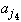| < M / 4, because both are in [M/2, M/4]
and in general, we see that
|-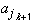| < M / 2k-1
because both are in an interval of length M / 2k-1. So, this proves that consecutive elements of this subsequence are close together. That is not enough, however, to say that the sequence is Cauchy, since for that not only consecutive elements must be close together, but all elements must get close to each other eventually.

So: take any> 0, and pick an integer N such that ???...??? (This trick is often used: first, do some calculation, then decide what the best choice for N should be. Right now, we have no way of knowing a good choice). Pretending, however, that we knew this choice of N, we continue the proof. For any k, m > N (with m > k) we have: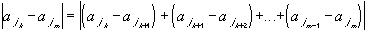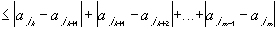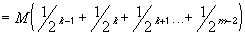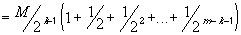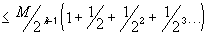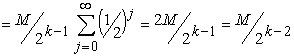Now we can see the choice for N: we want to make is so large, such that whenever k, m > N, the difference between the members of the subsequence is less than the prescribed. What is therefore the right choice for N to finish the proof ?Next | Previous | Glossary | Map